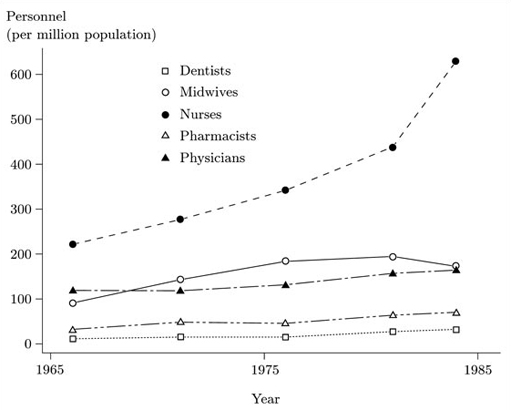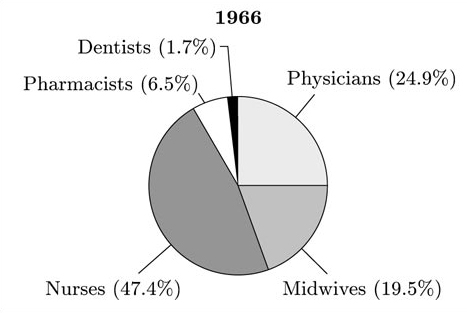Interpreting data: Boxplots and tables

This free course is available to start right now. Review the full course description and key learning outcomes and create an account and enrol if you want a free statement of participation.

Free course

3.2 Health care personnel in Thailand: activities

Activity 7 Health care personnel in Thailand: calculating percentages

Would it be helpful, in considering possible changes in the way health care personnel are divided into the five categories listed, to recalculate the numbers in the body of Table 3.1 as percentages either of the row totals or of the column totals? If you think it would be helpful, calculate the appropriate percentages and use the resulting table to comment on the data.

Recalculating the numbers in the body of the table as percentages of the totals of the columns would show clearly how the total number of health personnel in each year is divided between the five categories. It would not be very meaningful to calculate the percentages of the row totals. The results of calculating the numbers as percentages of the column totals are shown in Table 3.2.

Table 3.2 Health personnel in Thailand, 1966–1984 (percentages of column totals)

Category 1966 1971 1976 1981 1984
Physicians 25 20 18 18 15
Dentists 2 3 2 3 2
Pharmacists 6 8 6 7 6
Nurses 47 47 48 50 60
Midwives 20 24 26 22 16
Total (= 100%) 14512 20959 28571 38844 53096

These percentages show that the numbers for dentists and for pharmacists as percentages of the total health care personnel changed very little over the period covered by the table. However, the percentage of physicians fell reasonably steadily from 25% of the total in 1966 to 15% in 1984. The percentage of nurses grew slowly from 1966 to 1981, but then increased rapidly between 1981 and 1984. Finally, the percentage of midwives increased over the first ten years of the period covered, but then fell back to a level below the 1966 level. Of course, it should be borne in mind throughout that the total number of health care staff grew considerably over the period.

Your investigation in Activity 7 clarified the patterns in the original table; but it remains the case that the single most prominent feature of the table is the rise in total health care personnel over the period covered. However, it may well have occurred to you that the population served by these health care personnel also changed over the period in question. Thailand is, after all, a developing country that may well have experienced considerable population growth between 1966 and 1984.

In fact, estimates of the total population of Thailand in the years covered by Table 3.1 are also provided in the source from which that table was taken. They are given in Table 3.3.

Table 3.3 Estimated total population of Thailand, 1966–1984

1966 1971 1976 1981 1984
Population (millions) 31.1 35.4 40.3 44.9 50.7

These figures can be used to calculate the numbers of the different categories of health care personnel as a proportion of the total population. These proportions could, in principle at any rate, be shown as percentages, or as numbers per 100 000 population as in Example 2.1 (see especially Tables 2.6 and 2.7); but in this case they are clearer if shown as numbers per million population. The resulting proportions are given in Table 3.4. After reading through the table you should check that you understand how the numbers displayed were calculated.

Table 3.4 Health personnel per million population in Thailand, 1966–1984

Category 1966 1971 1976 1981 1984
Physicians 116.0 115.6 129.3 154.4 158.9
Dentists 8.1 15.0 14.9 23.5 26.2
Pharmacists 30.2 44.8 43.6 59.7 65.3
Nurses 221.1 275.7 340.0 436.5 627.8
Midwives 91.1 140.9 181.2 191.0 169.1
Total 466.6 592.1 709.0 865.1 1047.3

These calculations show clearly that, in relation to the size of the total population, the total numbers of health care personnel in Thailand rose considerably and steadily between 1966 and 1984. Putting it another way, the population rose over this period, but the numbers of health care personnel rose much faster. It would be reasonably straightforward to comment on the changes in the different categories in relation to total population on the basis of the numbers in Table 3.4, but this task can be made easier by drawing an appropriate graph.

Data like those in Table 3.4, where there is a value for each of a number of different times, are referred to as time series. (In this case, there are actually six different time series, one for each personnel category, plus one for the data on total health personnel.) A useful kind of graph for showing time series data is line plot. This is a scatterplot, with the times (years, in this case) along the horizontal axis and the actual data values of the time series along the vertical axis. It is conventional, for time series data, to join the resulting plots with straight line segments. This draws attention to the rate of change of the values in the series. For time series where there are large numbers of different time points, the symbols (for example, crosses or dots) representing the data points are often omitted for clarity. However, in these data there are only five time points so it is not necessary to do that. We could produce such line plots separately for each of the different categories, and indeed for the total. But it is often easier to compare the levels of different time series by plotting them all on the same graph. Figure 3.1 shows such a graph, with a set of points and a line for each of the categories. (The series for total personnel is omitted. Since the totals are necessarily bigger than the figures for the individual groups, including them on the same graph would squeeze up all the other lines towards the bottom of the diagram and make them hard to see.)Figure 3.1 Health care personnel per million population in Thailand

Activity 8 Health care personnel: interpreting line graphs

Comment on the main changes in health care personnel per million population in Thailand over the period 1966–1984, on the basis of Figure 3.1. Are there any important patterns in the data in Table 3.4 that the graph does not make clear?

It is clear from Figure 3.1 that the numbers for personnel in each category, in relation to the total population of the country, rose quite markedly over the period in question. The rise was very marked for nurses (who were the largest category throughout the period), particularly towards the end of the period covered, between 1981 and 1984. There are some exceptions to this overall pattern of increase, the most prominent of which is that the number of midwives per million population actually fell by considerable amount between 1981 and 1984.

It is very clear from the graph that the numbers for dentists and for pharmacists per million population are considerably less than the corresponding numbers for the other categories; and it is reasonably clear that the numbers for both these groups rose over the period. However, because the numbers for these groups are so much smaller than, say, the numbers for nurses, the lines for dentists and for pharmacists are rather squashed up at the bottom of the graph and it is therefore difficult to judge the size of the increase. It is thus much clearer from the table than from the graph that the number of dentists has more than tripled in relation to the population size over the period, and that the number of pharmacists has more than doubled. It is also not very clear from the graph that the numbers for dentists and for pharmacists fell slightly in relation to the population between 1971 and 1976.

Activity 9 Health care personnel: more on proportions

Your work in Activity 7, on the proportions of health care personnel in the different categories, did not take account of the total population of Thailand. Explain why, if you calculated the figures in Table 3.4 as percentages of the column totals, you would get exactly the same table of percentages as that in the solution to Activity 7. If you wanted to represent these percentages graphically, what kind of graph would you draw?

The figures in any particular column of Table 3.4, including the column totals, are equal to the figures in the corresponding column of Table 3.1 divided by the population estimate from the corresponding column of Table 3.3. Thus in carrying out a division to calculate a percentage from Table 3.4, the quantities in the numerator and in the denominator were calculated from Table 3.1 by dividing by the same population total. So the population total cancels out and the result of the division is identical to that obtained from Table 3.1.

One appropriate kind of graph that would draw particular attention to the proportions is the pie chart. We could draw separate pie charts for each of the years listed in the table. The appropriate pie charts for the 1966 and 1984 data are shown in Figures 3.2 and 3.3 respectively. (You were not asked to draw these in the activity!)Figure 3.2 Pie chart of health care personnel in Thailand in 1966Figure 3.3 Pie chart of health care personnel in Thailand in 1984

These pie charts draw particular attention to the large increase in the number of nurses as a proportion of total health care personnel in Thailand between 1966 and 1984.

In Example 3.1, the original data were counts of individuals. It proved useful to calculate appropriate percentages, by dividing the numbers in the body of the table by column totals. In Activity 2.4, you calculated similar percentages, but they were based on row totals. In Example 3.1, it would have made very little sense to calculate percentages of the row totals. However, in Activity 6, it would have made sense to calculate the percentages of column totals instead of row totals, but they would have provided information relevant to a different question from the one you were considering. For tables of counts in general, it is very often useful to calculate percentages of row totals, and/or of column totals, but it is important to think carefully about which set of percentages is informative in relation to the question you are interested in. The following example is intended to make this clear.

M248_2